Next: Restrictions on the Scenario Up: Initial Distributions of Binary Previous: Initial Distributions of Binary

## Star Formation Rate and Normalization of Stellar Populations

We have assumed a constant in time star formation rate and the total number of stars in the Galaxy,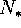, to be such that the total mass of the galaxy is; assuming the Salpeter mass functionĀ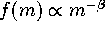, we get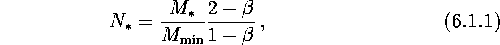where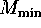stands for the minimum possible mass of the main sequence star. With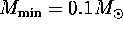,and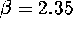, we obtain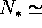2.6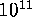stars in such a galaxy. This number agrees with a standard estimation of the galactic star formation rate of order ofper year. In each run of calculations, we trace the evolution ofbinaries with initial parameters randomly distributed according to the chosen initial laws. The evolution of each binary is calculated until either it comes to the stage where both components remain unchanged (say, double BH or BH with NS in a wide enough orbit that the orbital evolution due to the GR can be neglected), or until the time of evolution reaches a maximum value,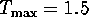yr.

We calculate the total number of systems,, passed through a given evolutionary stage ``i'', and calculate the sum of the stage durations,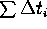. Then the total number of systems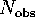at the given evolutionary stage simultaneously existing in the Milky Way is estimated asThe number of the initial binaries was taken high enough (typically 1 million each run) to produce a significant statistics (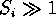) for every stage of interest.

Mike E. Prokhorov
Sat Feb 22 18:38:13 MSK 1997